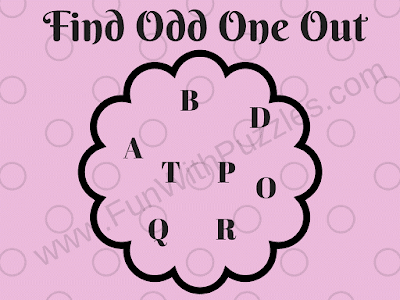Finding the Odd One Out is one of my favourite puzzle types. Odd One Out Puzzles are of many different types. It could be finding the Odd Picture Out picture among three similar-looking pictures. It also could be Finding Hidden Odd Picture among many different given similar pictures. Odd One Out Puzzles can also be with numbers. In Numbers Odd one Out puzzles, some numbers are given and one has to find the Odd or Different number which does not follow the same property as other numbers follow. There are many different Odd One Out puzzles on this website. These Odd One Out puzzles relate to finding the Odd English Letter among the given set of letters.
In each of the Odd One Out Puzzle pictures, there is a group of letters. These group of letters are related to each other with some specific property. However, there is one letter which does not follow this property and one has to find this Odd Letter Out.
These Odd One Out Puzzles range from Easy to Tough, with the first one being the easiest one and the difficulty level increased in each of the puzzles.1. Letters Odd One Out Easy Picture Puzzle2. Letters Odd One Out Kids Puzzle3. Letters Odd One Out Teens Puzzle4. Letters Odd One Out Hard Puzzle5. Letters Odd One Out Genius Puzzle

Do check out below listed Odd One Out Puzzles to test your observational, visual and analytic skills
List of Odd One Out Puzzles

1. Can you find Odd Letter Out?: These are the similar Odd Letter Out Picture Puzzles. However, puzzles here are tougher as compared to Odd Letter Out Puzzles listed in this post.

2. Fun Picture Puzzles for Kids with Answers:  These are very interesting Fun Odd One Out Picture Puzzles for kids in which one has to find which picture is different from the other three pictures.

3. Odd One Out Picture Brain teasers for kids: These are very much fun Odd One Out Picture Brain Teasers in which four pictures are given with each picture containing four different coloured circles. Three pictures out of these four pictures are easy and your challenge is to find the Odd One Out Picture.

4. Code Breaking Odd One Out Picture Puzzle with Answer: At last, there is a single Odd One Out Picture Puzzle in which different codes are given. Your challenge again here is to find this codes pattern which is different from other patterns.

------------------------
Here is the answer to 4th Brain Teaser Question of the post titled "Simple Math Riddles for Teens and Kids with Answers to Twist your Brain".
I have hidden this answer to avoid any spoiler. Do select the text below to read the answer.
-------------------